## 6. Frequency

Fluctuations in air pressure can occur at a wide range of rates. The listener interprets rapid changes in air pressure as high-pitched sound, and slower fluctuations as lower-pitched sound. In other words, the faster the air molecules move, the higher the perceived pitch.

Frequency is the rate of change in air pressure as measured by the number of cycles that occur per second.

For example, if a waveform repeats its complete cycle 300 times per second, its frequency is 300 cycles per second (abbreviated c.p.s.). Frequency is more commonly referred to in Hertz (abbreviated Hz); in this case, as 300 Hertz (or 300 Hz).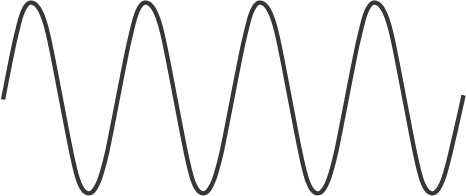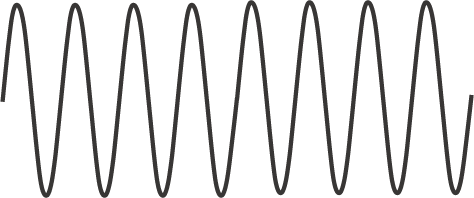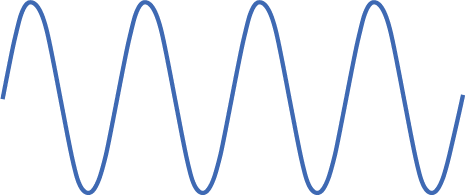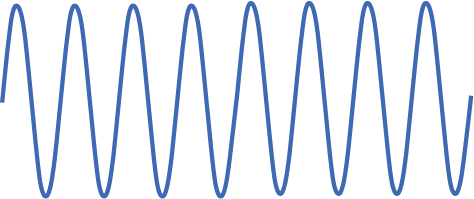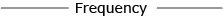Low Amplitude

Example 6-1: Adjusting the frequency of the waveform affects the pitch of the sound you hear.

Frequencies of 1000 Hz and above can be measured in kilohertz (abbreviated kHz). One kilohertz is equal to 1000 Hertz. Humans are capable of hearing frequencies between approximately 20 and 20,000 Hz; any frequency below 20 Hz is referred to as subaudio, while frequencies above 20 kHz are called ultrasonic.

The key aspect among this barrage of terminology is the correspondence between frequency and pitch. Frequency is a physical property of sound, while pitch is the listener's interpretation of frequency.